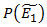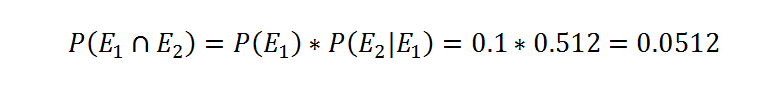# Bringing together stress testing and capital models – a Bayesian approach

Dan Georgescu & Manuel Sales.

Capital requirements for financial institutions are typically calculated using a statistical model and a risk measure such as VaR, whereas stress tests designed by regulators and risk managers are often based on subjective scenarios with no associated probability level. The stress test cannot therefore be easily linked to the capital measure. Taking insurance as an example, we show how to establish the link using intuitive tools which (i) respect the stress test designer’s intuition about causal direction, (ii) can be calibrated to pre-determined parameters such as correlations between risks, and (iii) can be easily communicated to and challenged by non-technical audiences.

Insurers hold capital for adverse events, calculated using a Value at Risk measure. (Part of the capital requirement of banks is also calculated using VaR, but we focus on insurers in this blog.) The Solvency II regulations, which will harmonize insurance capital requirements across Europe from January 2016 onwards, require firms to hold a Solvency Capital Requirement (SCR) such that there is a 99.5% probability that firms can meet their obligations to their policyholders over the following 12 months. Other provisions allow for the development of tools which assess the ability of firms to cope with possible events, e.g. stress tests; firms themselves are required to use stress tests and scenario analysis as part of their risk management framework. The question is how can we make sure that those stress tests validate or are at least consistent with the outputs of the model?  In other words, if a specified stress test results in a bigger loss than the SCR, how worried should management be?  Does this mean that the model has failed the validation test?  Or is it simply that the stress test was overly severe, perhaps with a confidence level greater than 99.5%? We show below how to integrate stress test design within the VaR framework. The proposed approach has further advantages: the direction of causation implicit in stress test design is respected, expert judgment about extreme events can be easily incorporated, and the final representation has a very intuitive presentation.

The Solvency II SCR allows for diversification effects between risks. Firms may calculate diversification using a correlation matrix between risks provided in a Standard Formula. Part of the Standard Formula correlations for market risk capital is as follows:Assuming that this correlation matrix is derived from a jointly Normal distribution for Equity, Property and Credit Spread risks, we can use it to inform the construction of a Bayesian network (BN) to generate coherent scenarios for stress testing. A BN is a directed graph where the nodes (representing risk categories in this case) are connected by arrows representing the direction of causation. Bayes’ theorem gives us a way to move between conditional, marginal and joint probabilities, and a Bayesian network automatically applies this theorem to all the variables in the tree. The practical set-up is similar to that suggested by Rebonato, with the exception that his risk manager does not have the luxury of a correlation matrix to get her started in the task of completing the BN. This allows additional flexibility for the risk manager to either specify causal relations in the construction of the BN, or to rely on the prior correlation matrix. It has been noted that associative measures of dependence, like correlations, do not capture the causal dependence that is implied in stress testing scenarios. In market risk terms, equity market falls cause implied volatility to rise, and not the other way around.

So we need to generate coherent scenarios – coherent in the sense that they respect the risk manager’s intuitions about causation but also comply with the correlation matrix, at least as a starting point. As we will demonstrate, such scenarios could be used to test the capital generated by capital models as they would be relevant to the calibration standard that they target.

For example, suppose that we want to investigate a scenario where the widening of credit spreads leads to an equity market crash, and these two drivers both cause a fall in commercial property prices. The challenge is to specify a scenario coherent with the correlations above.  Correlations are not very intuitive quantities (a detailed discussion of this point would be the subject of another article), so we may prefer to think in terms of conditional probabilities. A correlation of 50% between risks A and B in a framework where all risks have a joint Normal distribution means that if risk A is stressed to a 1-in-10 year event or beyond (equivalently, the 90th percentile of the risk A distribution or beyond), then there is a roughly 1-in-3 chance that risk B will also be stressed to that level. Similarly, a correlation of 75% is equivalent to a roughly 1-in-2 chance of seeing shocks at the 90th percentile or greater for risk B given a shock at that level for risk A.

Using these probabilities (derived from the Standard Formula correlations as above) we can quickly parameterise a BN as follows. Let E1 be the probability of a shock to risk 1, Credit Spreads. We know that P(E1) = 0.1, and therefore the probability of the complement event is= 0.9. From the correlation coefficients and the definition of a Gaussian copula (a copula is a tool for constructing multivariate distributions) we can calculate, along the lines of Sweeting and Fotiou’s coefficient of finite tail dependence, the probability of a 90th percentile shock to both Credit Spreads and Equity as:Therefore, the joint probabilities for E1 and E2 are calculated as follows:At this stage, the clear presentation of a table of probabilities means that senior management can already begin to challenge the calibration. Does it seem right that a 1-in-10 year Credit Spread widening and a 1-in-10 year Equity price fall happen about once in every 20 years?

Likewise, the following probabilities can be calculated from the correlation matrix, leading to the joint probabilities for E1, Eand E3:In words, the first line tells us that the probability of seeing a 1-in-10 year shock for each of Credit Spreads, Equity and Property is 2.7%. A stress test designed accordingly should be consistent with the outputs of the VaR model. It is worth spelling this out: the specification of this stress is coherent with the model correlation assumptions, and this tells us that 1-in-10 year stresses for Credit Spreads, Equity and Property happen together with probability of about 2%-3%.

Note that we could have obtained the table of joint probabilities above directly from the multivariate Normal assumption and correlation matrix. However, this presentation helps to show how we might go about parameterising a much bigger BN, where only some of the risks might be captured in the modelling framework.

Now that we have parameterised the BN, we show how to quickly investigate other questions like: what would be the revised probability of a Property market crash given that we have seen an Equity market crash? The prior answer was 10%, but conditioning on an Equity event the posterior probability of a Property crash calculated from our BN is about 50% (which we can verify by putting the numbers from above into the equation below, where P(E2) is the sum over the joint probabilities in the first four rows in the table above).Again, we can communicate this easily to a non-technical audience. For example, senior management would be in a position to challenge this result, perhaps by noting that 2 out of the last 3 equity crashes have also involved large property price falls and therefore asking whether this probability should be closer to 2-in-3.

We can also ask questions about what happens to the unconditional probabilities up the network: conditioning on an Equity event happening, the probability of having observed a Credit Spread widening also rises from 10% to about 50%. To use an everyday example: if it is raining, then the pavement is wet (reasoning with the direction of causation); however, if the pavement is wet, then we should also revise our probability that it was raining (reasoning from effect to cause). Again, management can consider whether this probability seems reasonable.

Further areas of analysis are suggested. For ease of presentation, we have used binary definitions of our risks: they either happen or they do not. But the tools presented allow continuous probability distributions to be used for each risk, thus bringing the stress test design even closer to the statistical copula models used in VaR calculation. We could  incorporate the risk exposure of a firm into the framework, to calculate directly the impact of such scenarios. Furthermore, BNs will deal much more easily with conditional cases, such as a node dictating whether we are in a stressed liquidity scenario or not, or a node dictating whether we are in a period of falling interest rates or rising interest rates.

In conclusion, we have shown how it is possible to easily parameterise a BN to build stress tests which are coherent with the assumptions of the capital model, which therefore allows us to build stress tests with an associated probability level.  We have, however, only scratched the surface of what is already possible: using BNs to add transparency to stress tests, reconcile stress testing with VaR and use the resulting framework to validate capital models.

Dan Georgescu and Manuel Sales work in the Bank’s Life Insurance Division.

If you want to get in touch, please email us at bankunderground@bankofengland.co.uk

Bank Underground is a blog for Bank of England staff to share views that challenge – or support – prevailing policy orthodoxies. The views expressed here are those of the authors, and are not necessarily those of the Bank of England, or its policy committees.